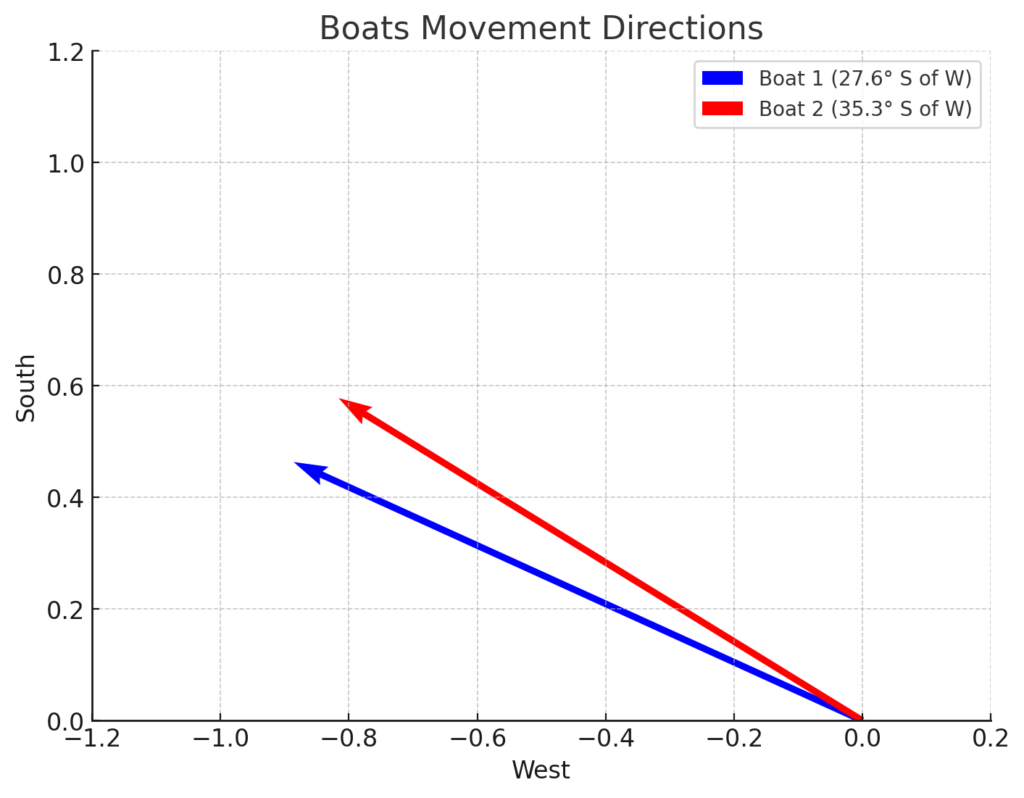#Two racing boats set out from the same dock and speed away at the same constant speed of 101 km/h for half an hour (0.5 hr). Boat 1 is headed 27.6° south of west, and Boat 2 is headed 35.3° south of west, as shown in the graph above. During this half-hour calculate: How much farther west does Boat 1 travel, compared to Boat 2 in kilometers. How much farther south does Boat 2 travel, compared to the Boat 2 in kilometers.

1. .98 km
2. 1.61 km

## Written By Prof Phy AI

Step Formula / Calculation Reasoning
1 v = 101 , \text{km/h}, t = 0.500 , \text{hr} Defining the constant speed and time for both boats.
2 v_{\text{m/s}} = \frac{101 \times 1000}{3600} Converting the speed from km/h to m/s.
3 v_{\text{west, boat 1}} = v_{\text{m/s}} \cos(\theta_{\text{boat 1}}), v_{\text{south, boat 1}} = v_{\text{m/s}} \sin(\theta_{\text{boat 1}}) Decomposing Boat 1’s speed into westward and southward components.
4 v_{\text{west, boat 2}} = v_{\text{m/s}} \cos(\theta_{\text{boat 2}}), v_{\text{south, boat 2}} = v_{\text{m/s}} \sin(\theta_{\text{boat 2}}) Decomposing Boat 2’s speed into westward and southward components.
5 \text{Distance west, boat 1} = v_{\text{west, boat 1}} \times t, \text{Distance south, boat 1} = v_{\text{south, boat 1}} \times t Calculating the westward and southward distances for Boat 1.
6 \text{Distance west, boat 2} = v_{\text{west, boat 2}} \times t, \text{Distance south, boat 2} = v_{\text{south, boat 2}} \times t Calculating the westward and southward distances for Boat 2.
7 \text{Difference west} = \text{Distance west, boat 1} – \text{Distance west, boat 2} Calculating the difference in the westward distance between the two boats.
8 \text{Difference south} = \text{Distance south, boat 2} – \text{Distance south, boat 1} Calculating the difference in the southward distance between the two boats.
9 \text{Difference west} \approx 0.98 km, \text{Difference south} \approx 1.61 km Evaluating the differences in distances.

During the half-hour journey, Boat 1 travels approximately 0.98 km farther west compared to Boat 2, and Boat 2 travels approximately 1.61 km farther south compared to Boat 1.

## Discover how students preformed on this question | Coming Soon

###### Login to Join the Discussion
1. .98 km
2. 1.61 km## Suggest an Edit

##### Nerd-Notes.com
KinematicsForces
\Delta x = v_i \cdot t + \frac{1}{2} a \cdot t^2F = m \cdot a
v = v_i + a \cdot tF_g = \frac{G \cdot m_1 \cdot m_2}{r^2}
a = \frac{\Delta v}{\Delta t}f = \mu \cdot N
R = \frac{v_i^2 \cdot \sin(2\theta)}{g}
Circular MotionEnergy
F_c = \frac{m \cdot v^2}{r}KE = \frac{1}{2} m \cdot v^2
a_c = \frac{v^2}{r}PE = m \cdot g \cdot h
KE_i + PE_i = KE_f + PE_f
MomentumTorque and Rotations
p = m \cdot v\tau = r \cdot F \cdot \sin(\theta)
J = \Delta pI = \sum m \cdot r^2
p_i = p_fL = I \cdot \omega
Simple Harmonic Motion
F = -k \cdot x
T = 2\pi \sqrt{\frac{l}{g}}
T = 2\pi \sqrt{\frac{m}{k}}
ConstantDescription
gAcceleration due to gravity, typically 9.8 , \text{m/s}^2 on Earth’s surface
GUniversal Gravitational Constant, 6.674 \times 10^{-11} , \text{N} \cdot \text{m}^2/\text{kg}^2
\mu_k and \mu_sCoefficients of kinetic (\mu_k) and static (\mu_s) friction, dimensionless. Static friction (\mu_s) is usually greater than kinetic friction (\mu_k) as it resists the start of motion.
kSpring constant, in \text{N/m}
VariableSI Unit
s (Displacement)\text{meters (m)}
v (Velocity)\text{meters per second (m/s)}
a (Acceleration)\text{meters per second squared (m/s}^2\text{)}
t (Time)\text{seconds (s)}
m (Mass)\text{kilograms (kg)}
VariableDerived SI Unit
F (Force)\text{newtons (N)}
E, PE, KE (Energy, Potential Energy, Kinetic Energy)\text{joules (J)}
P (Power)\text{watts (W)}
p (Momentum)\text{kilogram meters per second (kg·m/s)}
\tau (Torque)\text{newton meters (N·m)}
I (Moment of Inertia)\text{kilogram meter squared (kg·m}^2\text{)}
f (Frequency)\text{hertz (Hz)}

General Metric Conversion Chart

Example of using unit analysis: Convert 5 kilometers to millimeters.

1. Start with the given measurement: \text{5 km}

2. Use the conversion factors for kilometers to meters and meters to millimeters: \text{5 km} \times \frac{10^3 \, \text{m}}{1 \, \text{km}} \times \frac{10^3 \, \text{mm}}{1 \, \text{m}}

3. Perform the multiplication: \text{5 km} \times \frac{10^3 \, \text{m}}{1 \, \text{km}} \times \frac{10^3 \, \text{mm}}{1 \, \text{m}} = 5 \times 10^3 \times 10^3 \, \text{mm}

4. Simplify to get the final answer: \boxed{5 \times 10^6 \, \text{mm}}

Prefix

Symbol

Power of Ten

Equivalent

Pico-

p

10^{-12}

Nano-

n

10^{-9}

Micro-

µ

10^{-6}

Milli-

m

10^{-3}

Centi-

c

10^{-2}

Deci-

d

10^{-1}

(Base unit)

10^{0}

Deca- or Deka-

da

10^{1}

Hecto-

h

10^{2}

Kilo-

k

10^{3}

Mega-

M

10^{6}

Giga-

G

10^{9}

Tera-

T

10^{12}

1. Some answers may be slightly off by 1% depending on rounding, etc.
2. Answers will use different values of gravity. Some answers use 9.81 m/s2, and other 10 m/s2 for calculations.
3. Variables are sometimes written differently from class to class. For example, sometime initial velocity v_i is written as u ; sometimes \Delta x is written as s .
4. Bookmark questions that you can’t solve so you can come back to them later.
5. Always get help if you can’t figure out a problem. The sooner you can get it cleared up the better chances of you not getting it wrong on a test!